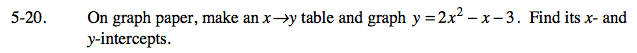Home > AC > Chapter 5 > Lesson 5.1.2 > Problem5-20

5-20.

On graph paper, make an x y table and graph y = 2x2x − 3. Find its x- and y-intercepts. Homework Help ✎When making your table, use integer values from −2 to 2 for x.

Be sure to use parentheses and follow the Order of Operations.

For example, if x = −2, then y = 2(−2)2 − (−2) − 3 = 2(4) + 2 − 3 = 7.